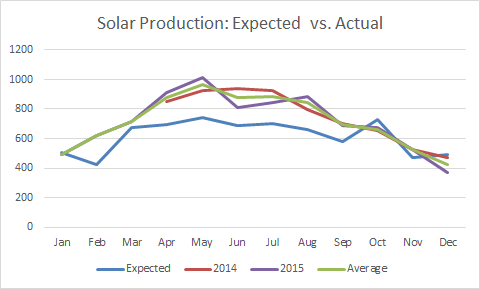Here are my solar power production numbers for November – 526 kWh (at inverter). 523 kWh (at Dominion meter). PVWatts estimate was 473 kWh.

Here are my solar power production numbers for December – 372 kWh (at inverter). 372 kWh (at Dominion meter). PVWatts estimate was 490 kWh.